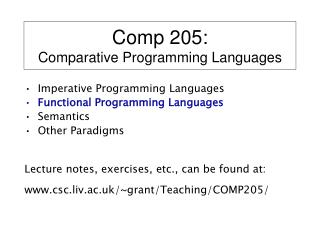DownloadDownload PresentationComp 205: Comparative Programming Languages

# Comp 205: Comparative Programming Languages

Télécharger la présentation## Comp 205: Comparative Programming Languages

- - - - - - - - - - - - - - - - - - - - - - - - - - - E N D - - - - - - - - - - - - - - - - - - - - - - - - - - -
##### Presentation Transcript

1. Comp 205:Comparative Programming Languages • Imperative Programming Languages • Functional Programming Languages • Semantics • Other Paradigms • Lecture notes, exercises, etc., can be found at: • www.csc.liv.ac.uk/~grant/Teaching/COMP205/

2. Functional Languages • Today, we will look at: • Functions • Types • Languages (primarily Haskell)

3. Levels of Abstraction • Hardwiring (logic gates) • Machine code (chip-set instructions) • Assembly language • "High-level" languages(Fortran, Algol, Cobol, Pascal, Ada, Modula) • Object-oriented languages(Simula67, Smalltalk, Java) • Declarative languages • … • Specification languages (OBJ, Z)

4. Code Generation Gaps "Select all items in an integer array that are less than 10" j := 1; FOR i := 1 TO LineLength DO IF line[i] < 10 THEN newline[j] := line[i]; j := j + 1; END END From Chris Clack et al., Programming with Miranda. Prentice Hall, London 1995.

5. Declarative Languages • Declarative Languages can be divided into • Functionaland Logic languages. • Functional languages include: • LISP • SML • Haskell • Hope • Logic languages include • Prolog • Eqlog

6. What is a Function? • y = 2 * x • x  2 * x • twice(x) = 2 * x • twice x = 2 * x (Prefix Notation)

7. Some Terminology twice x = 2 * x Function name Formal parameter Function body

8. Formal Parameters • Formal parameters can be renamed: • twice x = 2 * x • twice z = 2 * z • twice i = 2 * i

9. Formal Parameters Again Formal parameters can be renamed: public static int twice(int x) { return 2 * x ; } is the same as: public static int twice(int i) { return 2 * i ; }

10. Actual Parameters • Function evaluation works by substituting an • actual parameter for a formal parameter: • twice x = 2 * x • twice 7 = 2 * 7 = 14

11. Actual Parameters Again The same kind of evaluation through substitution is at work in imperative languages: public static int twice(int x) { return 2 * x ; } ... int i = twice(7); // i = 14

12. Programming with Functions Functional programming languages allow direct definitions of what is to be computed (rather than the how of imperative programming languages) twice x = 2 * x

13. Functions and Types I • Functions need not be restricted to numbers; • they can work on any sort of data. • Among the data structures of interest to • Computer Science are: • boolean values (true, false) • characters • strings • lists • trees • graphs

14. Functions and Types II The type of a function refers to the kind of argument it takes, and the kind of result it returns. The function twice takes a number as argument and returns a number as result; its type is: num  num Or: twice : num  num

15. Functional Programming Languages The example twice is misleading, because its definition looks algorithmic. Not all functions are as easy to express in a programming language….

16. The Fibonacci Sequence 1, 1, 2, 3, 5, 8, 13, 21, 34, 55, 89, …. Each number in this sequence is the sum of the two preceding numbers (apart from the first two). fib 0 = 1 fib 1 = 1 fib n = (fib (n-2)) + (fib (n-1)), if n > 1

17. The Fibonacci Sequence (Java remix) public static int fib(int n) { int i = 0; int x = 1; int y = 1; while (i < n) { y += x; x = y - x; i++; } return x; }

18. The Fibonacci Sequence (Haskell remix) fib n = fib1 where (fib1, fib2) = fibs n fibs 0 = (1,1) fibs n = (f2, f1 + f2) where (f1,f2) = fibs (n-1), if n > 0

19. Factorials The factorial of a number n is the product of all the numbers from 1 to n : fact n = 1 * 2 * ••• * n However, this can be defined recursively: fact 0 = 1 fact n = (fact (n-1)) * n

20. Summary • Functions work by substitution and evaluation • Functions can have types • Functional programming languages also workby substitution and evaluation • Functional programming languages emphasisewhat is to be computed, rather than how • Next: Haskell